Computer Algebra I: Mathematica, SymPy, Sage, Maxima

a side-by-side reference sheet

mathematica sympy sage maxima
symbolic expressions
mathematica sympy sage maxima
literal expr = 1 + x + x^2 x = symbols('x')

expr = 1 + x + x^2
expr = 1 + x + x^2 expr = 1 + x + x^2;
prevent simplification HoldForm[x + x]
x + x // HoldForm
variable update expr = 1 + x
x = 3
(* 4: *)
expr
x = symbols('x')
expr = 1 + x
x = 3
# 1 + x:
expr
expr = 1 + x
x = 7
# 1 + x:
expr
expr: 1 + x;
x: 3;
/* 1 + x: */
expr;
substitute (* {3, 3}: *)
ReplaceAll[{x, x}, x -> 3]

(* {3, 3}: *)
{x, x} /. x -> 3

(* {3, 4}: *)
{x, y} /. {x -> 3, y -> 4}
Matrix([x, x]).subs(x, 3) vector([x, x]).subs({x: 3}) /* [3, 3]: */
subst(3, x, [x, x]);
piecewise-defined expression Piecewise[{{x, x >= 0}, {-x, x < 0}}]

(* otherwise case: *)
Piecewise[{{-x, x < 0}}, x]
Piecewise((-x, x < 0), (x, x >= 0))

# otherwise case:
Piecewise((-x, x < 0), (x, True))
piecewise([
((-infinity,0), -x),
((0,infinity), x)])
if x < 0 then -x else x;

/* integrating over piecewise-defined expression fails */
simplify Simplify[Cos[x]^2 + Sin[x]^2]

(* perform more simplications: *)
FullSimplify[-(1/2) I E^(-I x) (-1 + E^(2 I x))]
simplify(cos(x)**2 + sin(x)**2)
assumption Simplify[Sqrt[x^2], Assumptions -> x >= 0]
Simplify[(-1)^(n * (n + 1)),
Assumptions -> Element[n, Integers]]

(* perform fewer simplications: *)
Refine[Sqrt[x^2], Assumptions -> x >= 0]
Refine[(-1)^(n * (n + 1)), Element[n, Integers]]
x = symbols('x', positive=True)
sqrt(x ** 2)

n = symbols('n', integer=True)
(-1)**((n) * (n + 1))
assume(x > 0)
sqrt(x^2)
assume(x > 0);
sqrt(x^2);

/* There is no assumption predicate for
integer variables. */
assumption predicates Element[x, Complexes]
Element[x, Reals]
Element[x, Algebraics]
Element[x, Rationals]
Element[x, Integers]
Element[x, Primes]
Element[x, Integers] && Mod[x, 5] == 0
Element[x, Booleans]

(* assumptions can use inequalities and logical operators: *)
x > 0 || x < 0
# a partial list:
complex
real
algebraic
rational
integer
positive
nonpositive
negative
nonnegative
nonzero
prime
odd
even
assume(x, 'complex')
assume(x, 'real')
assume(x, 'rational')
assume(x, 'integer')
assume(x, 'odd')
assume(x, 'even')
Assumptions can only be created using relational operators.
list assumptions None. Assumptions are always local. x.assumptions0 assumptions() facts(x);

# assumptions on all symbols:
facts();
remove assumption None. Assumptions are always local. # removes all assumptions about x:
x = symbols('x')
forget(x > 0)

# rm all assumptions:
forget()
forget(x > 0);
calculus
mathematica sympy sage maxima
limit

Limit[Sin[x]/x, x -> 0] limit(sin(x)/x, x, 0) limit(sin(x)/x, x=0) limit(sin(x)/x, x, 0);
limit at infinity

Limit[1/x, x -> Infinity] limit(1/x, x, oo) limit(1/x, x=infinity) limit(1/x, x, inf);
one-sided limit

from left, from right
Limit[1/x, x -> 0, Direction -> 1]
Limit[1/x, x -> 0, Direction -> -1]
limit(1/x, x, 0, '-')
limit(1/x, x, 0, '+')
limit(1/x, x=0, dir='-')
limit(1/x, x=0, dir='+')
limit(1/x, x, 0, minus);
limit(1/x, x, 0, plus);
derivative D[x^3 + x + 3, x]

D[x^3 + x + 3, x] /. x -> 2
diff(x**3 + x + 3, x)

diff(x**3 + x + 3, x).subs(x, 2)
diff(x^3 + x + 3, x)

diff(x^3 + x + 3, x).subs({x: 2})

# derivative is synonym of diff
diff(x^3 + x + 3, x);

at(diff(x^3 + x + 3, x), [x=2]);
derivative of a function f[x_] = x^3 + x + 3

(* returns expression: *)
D[f[x, x]]

(* return functions: *)
f'
Derivative[f]

(* evaluating derivative at a point: *)
f'
Derivative[f]
f(x) = x^3 + x + 3

diff(f)

diff(f)(2)
constants (* a depends on x; b does not: *)
D[a x + b, x, NonConstants -> {a}]

Dt[a x + b, x, Constants -> {b}]
/* symbols constant unless declared with depends: */
depends(a, x);
diff(a * x + b, x);

/* makes a constant again: */
remove(a, dependency);
higher order derivative D[Log[x], {x, 3}]
Log'''[x]
Derivative[Log][x]
diff(log(x), x, 3) diff(log(x), x, 3) diff(log(x), x, 3);
mixed partial derivative D[x^9 * y^8, x, y, y]
D[x^9 * y^8, x, {y, 2}]
diff(x**9 * y**8, x, y, y) diff(x^9 * y^8, x, 1).diff(y, 2) diff(x^9 * y^8, x, 1, y, 2);
div, grad, and curl Div[{x^2, x * y, x * y * z}, {x, y, z}]

Grad[2 * x * y * z^2, {x, y, z}]

Curl[{x * y * z, y^2, 0}, {x, y, z}]
antiderivative

Integrate[x^3 + x + 3, x] integrate(x**3 + x + 3, x) integral(x^3 + x + 3, x) integrate(x^3 + x + 3, x);
definite integral

Integrate[x^3 + x + 3, {x, 0, 1}] integrate(x**3 + x + 3, [x, 0, 1]) integral(x^3 + x + 3, x, 0, 1) integrate(x^3 + x + 3, x, 0, 1);
improper integral

Integrate[Exp[-x], {x, 0, Infinity}] integrate(exp(-x), (x, 0, oo)) integral(exp(-x), x, 0, infinity) integrate(exp(-x), x, 0, inf);
double integral (* integrates over y first: *)
Integrate[x^2 + y^2, {x, 0, 1}, {y, 0, x}]
f = integrate(x**2 + y**2, (y, 0, x))
integrate(f, (x, 0, 1))
integral(integral(x^2+y^2, y, 0, x), x, 0, 1) integrate(
integrate(x^2+y^2, y, 0, x), x, 0, 1);
find poles

residue

Residue[1/(z - I), {z, I}] residue(1/(z-I), z, I) f(z) = 1/(z - I)
f.maxima_methods().residue(z, I)
residue(1 / (z - %i), z, %i);
sum

Sum[2^i, {i, 1, 10}] Sum(2**i, (i, 1, 10)).doit() sum(2^i for i in (1..10)) sum(2^i, i, 1, 10);
series sum

Sum[2^-n, {n, 1, Infinity}] Sum(2**(-n), (n, 1, oo)).doit() sum(2^-n, n, 1, infinity) sum(2^-n, n, 1, inf), simpsum;
series expansion of function Series[Cos[x], {x, 0, 10}] series(cos(x), x, n=11) taylor(cos(x), x, 0, 10) taylor(cos(x), [x, 0, 10]);
omitted order term expr = 1 + x + x/2 + x^2/6 + O[x]^3

(* remove omitted order term: *)
Normal[expr]
product

Product[2*i + 1, {i, 0, 9}] Product(2*i + 1, (i, 0, 9)).doit() prod(2*i + 1 for i in (0..9)) product(2*i + 1, i, 0, 9);
equations and unknowns
mathematica sympy sage maxima
solve equation

Solve[x^3 + x + 3 == 0, x] solve(x**3 + x + 3, x) solve(x^3 + x + 3 == 0, x) solve(x^3 + x + 3 = 0, x);
solve equations Solve[x + y == 3 && x == 2 * y,
{x, y}]

(* or: *)
Solve[{x + y == 3, x == 2 * y}, {x, y}]
solve([x + y - 3, 3*x - 2*y], [x, y]) solve([x + y == 3, x == 2*y], x, y) solve([x + y = 3, x = 2*y], [x, y]);
differential equation DSolve[y'[x] == y[x], y[x], x] y = Function('y')

dsolve(Derivative(y(x), x) - y(x), y(x))
y = function('y')(x)

desolve(diff(y, x) == y, y)
desolve([diff(y(x), x) = y(x)], [y(x)]);
differential equation with boundary condition DSolve[{y'[x] == y[x], y == 1}, y[x], x]

DSolve[{y''[x] == y[x], y == 1, y' == 2},
y[x], x]
support for boundary conditions is limited y = function('y')(x)

# y(0) = 1:
desolve(diff(y, x) == y, y, [0, 1])

# y(0) = 1 and y'(0) = 2:
desolve(diff(y, x, x) == y, y, [0, 1, 2])
atvalue(y(x), x=0, 1);
desolve([diff(y(x), x) = y(x)], [y(x)]);
differential equations eqn1 = x'[t] == x[t] - x[t] * y[t]
eqn2 = y'[t] == x[t] * y[t] - y[t]
DSolve[{eqn1, eqn2}, {x[t], y[t]}, t]
eqn1: diff(x(t), t) = x(t) - x(t) * y(t);
eqn2: diff(y(t), t) = x(t) * y(t) - y(t);
desolve([eqn1, eqn2], [x(t), y(t)]);
recurrence equation eqns = {a[n + 2] == a[n + 1] + a[n],
a == 0,
a == 1}

RSolve[eqns, a[n], n]

(* remove Fibonacci[] from solution: *)
FunctionExpand[RSolve[eqns, a[n], n]]
n = symbols('n')
a = Function('a')
eqn = a(n+2) - a(n+1) - a(n)

rsolve(eqn, a(n), {a(0): 0, a(1): 1})
solve_rec(a[n]=a[n-1]+a[n-2], a[n], a = 0, a = 1);
optimization
mathematica sympy sage maxima
minimize (* returns list of two items: min value and rule
transforming x to argmin *)

Minimize[x^2 + 1, x]

(* 2 ways to get min value: *)
Minimize[x^2 + 1, x][]
MinValue(x^2 + 1, x]

(* 2 ways to get argmin: *)
x /. Minimize[x^2 + 1, x][]
ArgMin[x^2 + 1, x]
maximize Maximize[-x^4 + 3 x^3, x]

Maxvalue[-x^4 + 3 x^3, x]
ArgMax[-x^4 + 3 x^3, x]
objective with unknown parameter (* minval and argmin are expressions
containing a: *)

Minimize[(x - a)^2 + x, x]
unbounded behavior (* MaxValue will be Infinity; MinValue will be
-Infinity *)
multiple variables (* returns one solution: *)
Minimize[x^4 - 2 x^2 + 2 y^4 - 3 y^2, {x, y}]
constraints Minimize[{-x - 2 y^2, y^2 <= 17, 2 x + y <= 5},
{x, y}]
infeasible behavior (* MaxValue will be -Infinity; MinValue will be
Infinity; ArgMax or ArgMin will be
Indeterminate *)
integer variables Maximize[{x^2 + 2*y,
x >= 0, y >= 0,
2 x + Pi * y <= 4},
{x, y}, Integers]
vectors
mathematica sympy sage maxima
vector literal (* row vector is same as array: *)
{1, 2, 3}
# column vector:
Matrix([1, 2, 3])
vector([1, 2, 3]) /* row vector is same as array: */
[1, 2, 3];
constant vector

all zeros, all ones
Table[0, {i, 1, 100}]
Table[1, {i, 1, 100}]
Matrix( * 100)
Matrix( * 100)
vector( * 100)
vector( * 100)
makelist(0, 100);
makelist(1, 100);
vector coordinate (* indices start at one: *)
{6, 7, 8}[]
Matrix([6, 7, 8]) vector([6, 7, 8]) [6, 7, 8];
vector dimension

Length[{1, 2, 3}] len(Matrix([6, 7, 8]))
Matrix([6, 7, 8]).shape
len(vector([1, 2, 3])) length([1, 2, 3]);
element-wise arithmetic operators + - * /
+ -

# element-wise multiplication:
A = Matrix([1, 2, 3])
B = Matrix([2, 3, 4])
A.multiply_elementwise(B)
+ - + - * /
vector length mismatch

error raises ShapeError raises TypeError error
scalar multiplication 3 {1, 2, 3}
{1, 2, 3} 3
* may also be used
3 * Matrix([1, 2, 3])
Matrix([1, 2, 3]) * 3
3 * vector([1, 2, 3])
vector([1, 2, 3]) * 3
3 * [1, 2, 3];
[1, 2, 3] * 3;
dot product {1, 1, 1} . {2, 2, 2}
Dot[{1, 1, 1}, {2, 2, 2}]
v1 = Matrix([1, 1, 1])
v2 = Matrix([2, 2, 2])
v1.dot(v2)
vector([1, 1, 1]) * vector([2, 2, 2])
vector([1,1,1]).dot_product(vector([2,2,2]))
[1, 1, 1] . [2, 2, 2];
cross product Cross[{1, 0, 0}, {0, 1, 0}] e1 = Matrix([1, 0, 0])
e2 = Matrix([0, 1, 0])
e1.cross(e2)
e1 = vector([1, 0, 0])
e2 = vector([0, 1, 0])
e1.cross_product(e2)
norms Norm[{1, 2, 3}, 1]
Norm[{1, 2, 3}]
Norm[{1, 2, 3}, Infinity]
vec = Matrix([1, 2, 3])

vec.norm(1)
vec.norm()
vec.norm(inf)
vector([1, 2, 3]).norm(1)
vector([1, 2, 3]).norm()
vector([1, 2, 3]).norm(infinity)
orthonormal basis Orthogonalize[{{1, 0, 1}, {1, 1, 1}}] A = matrix([[1, 0, 1], [1, 1, 1]]

# Rows of B are orthogonal and span same
# space as rows of A. 2nd return value
# expresses rows of A as linear combos
# of rows of B.

B, _ = A.gram_schmidt()

gramschmidt([[1, 0, 1], [1, 1, 1]]);
matrices
mathematica sympy sage maxima
literal or constructor (* used a nested array for each row: *)
{{1, 2}, {3, 4}}

(* display as grid with aligned columns: *)
MatrixForm[{{1, 2}, {3, 4}}]
Matrix([[1, 2], [3, 4]]) matrix([[1, 2], [3, 4]]) matrix([1, 2], [3, 4]);
construct from sequence ArrayReshape[{1, 2, 3, 4, 5, 6}, {2, 3}] Matrix(2, 3, [1, 2, 3, 4, 5, 6]) matrix([1, 2, 3, 4, 5, 6], nrows=2)
constant matrices

all zeros, all ones
Table[0, {i, 3}, {j, 3}]
Table[1, {i, 3}, {j, 3}]
zeros(3, 3)
ones(3, 3)
matrix( * 9, nrows=3)
matrix( * 9, nrows=3)
zeromatrix(3, 3);

f[i, j] := 1;
genmatrix(f, 3, 3);
diagonal matrices
and identity
DiagonalMatrix[{1, 2, 3}]
IdentityMatrix
diag(*[1, 2, 3])
eye(3)
diag = [1, 2, 3]
d = {(i, i): v for (i, v) in enumerate(diag)}
Matrix(3, 3, d)

matrix.identity(3)
ident(3) * [1, 2, 3];
ident(3);
matrix by formula Table[1/(i + j - 1), {i, 1, 3}, {j, 1, 3}] Matrix(3, 3, lambda i, j: 1/(i + j + 1)) h2[i, j] := 1/(i + j -1);
genmatrix(h2, 3, 3);
dimensions (* returns {3, 2}: *)
Dimensions[{{1, 2}, {3, 4}, {5, 6}}]
A = matrix([[1, 2], [3, 4], [5, 6]])

# returns (3, 2):
A.shape
A = matrix([[1, 2], [3, 4], [5, 6]])
A.nrows()
A.ncols()
A: matrix([1, 2, 3], [4, 5, 6]);
matrix_size(A);
element lookup (* top left corner: *)
{{1, 2}, {3, 4}}[[1, 1]]
A = Matrix([[1, 2], [3, 4]])

# top left corner:
A[0, 0]
A = matrix([[1, 2], [3, 4]])
A[0, 0]
A
A: matrix([1, 2], [3, 4]);

A[1, 1];
A;
extract row (* first row: *)
{{1, 2}, {3, 4}}[]
# first row:
A[0, :]
# first row as vector:
A
A.rows()
row(matrix([1, 2], [3, 4]), 1);
matrix([1, 2], [3, 4]);
extract column (* first column as array: *)
{{1, 2}, {3, 4}}[[All, 1]]
# first column as 1x2 matrix:
A[:, 0]
# first column as vector:
A.columns()
col(matrix([1, 2], [3, 4]), 1);
extract submatrix A = {{1, 2, 3}, {4, 5, 6}, {7, 8, 9}}
A[[1;;2, 1;;2]]
rows = [[1, 2, 3], [4, 5, 6], [7, 8, 9]]
A = Matrix(rows)
A[0:2, 0:2]
A = matrix(range(1, 10), nrows=3)

# takes two lists of indices:
A.matrix_from_rows_and_columns([0, 1], [0, 1])
scalar multiplication 3 * {{1, 2}, {3, 4}}
{{1, 2}, {3, 4}} * 3
3 * Matrix([[1, 2], [3, 4]])
Matrix([[1, 2], [3, 4]]) * 3
3 * matrix([[1, 2], [3, 4]])
matrix([[1, 2], [3, 4]]) * 3
3 * matrix([1, 2], [3, 4]);
matrix([1, 2], [3, 4]) * 3;
element-wise operators + - * /
+ -

A.multiply_elementwise(B)
+ - + - * /
product A = {{1, 2}, {3, 4}}
B = {{4, 3}, {2, 1}}
Dot[A, B]
(* or use period: *)
A . B
A = matrix([[1, 2], [3, 4]])
B = matrix([[4, 3], [2, 1]])
A * B
A = matrix([[1, 2], [3, 4]])
B = matrix([[4, 3], [2, 1]])
A * B
A: matrix([1, 2], [3, 4]);
B: matrix([4, 3], [2, 1]);
A . B;
multiply by vector {{1, 2}, {3, 4}} . {7, 8}
Dot[{{1, 2}, {3, 4}}, {5, 6}]
matrix([[1, 2], [3, 4]]) * vector([5, 6]) matrix([1, 2], [3, 4]) . transpose([5, 6]);
power MatrixPower[{{1, 2}, {3, 4}}, 3]

(* element-wise operator: *)
A ^ 3
A ** 3 A ^ 3
A ** 3
matrix([1, 2], [3, 4]) ^^ 3;
exponential MatrixExp[{{1, 2}, {3, 4}}] exp(matrix([[1, 2], [3, 4]]))
log MatrixLog[{{1, 2}, {3, 4}}]
kronecker product A = {{1, 2}, {3, 4}}
B = {{4, 3}, {2, 1}}
KroneckerProduct[A, B]
A = matrix([[1, 2], [3, 4]])
B = matrix([[4, 3], [2, 1]])
A.tensor_product(B)
A: matrix([1, 2], [3, 4]);
B: matrix([4, 3], [2, 1]);
kronecker_product(A, B);
norms A = {{1, 2}, {3, 4}}

Norm[A, 1]
Norm[A, 2]
Norm[A, Infinity]
Norm[A, "Frobenius"]
A = matrix([[1, 2], [3, 4]])

# floating point values:
A.norm(1)
A.norm()
A.norm(infinity)
A.norm('frob')
A: matrix([1, 2], [3, 4]);

mat_norm(A, 1);
/* none */
mat_norm(A, inf);
mat_norm(A, frobenius);
transpose Transpose[{{1, 2}, {3, 4}}]

(* or ESC tr ESC for T exponent notation *)
A.T A.transpose() transpose(A);
conjugate transpose A = {{1, I}, {2, -I}}
ConjugateTranspose[A]

(* or ESC ct ESC for dagger exponent notation *)
M = Matrix([[1, I], [2, -I]])
M = matrix([[1, I], [2, -I]])
M.conjugate_transpose()
ctranspose(matrix([1, %i], [2, -%i]));
inverse Inverse[{{1, 2}, {3, 4}}]

(* expression left unevaluated: *)
Inverse[{{1, 0}, {0, 0}}]
A.inv()

# raises ValueError:
Matrix([[1, 0], [0, 0]]).inv()
A.inverse()
A ^ -1
A ** -1
invert(A);
A ^^ -1;

/* error: */
invert(matrix([1, 0], [0, 0]));
row echelon form RowReduce[{{1, 1}, {1, 1}}] matrix([[1, 1], [1, 1]]).echelon_form() echelon(matrix([1, 1], [1, 1]));
pseudoinverse PseudoInverse[{{1, 0}, {3, 0}}]
determinant Det[{{1, 2}, {3, 4}}] A.det() A.determinant() determinant(A);
trace Tr[{{1, 2}, {3, 4}}] A.trace() load("nchrpl");

mattrace(matrix([1, 2], [3, 4]));
characteristic polynomial CharacteristicPolynomial[{{1, 2}, {3, 4}}, x] matrix([[1, 2], [3, 4]]).charpoly('x') A: matrix([1, 2], [3, 4]);

charpoly(A, x);

minimalPoly(jordan(ident(3)));
rank MatrixRank[{{1, 1}, {0, 0}}] matrix([[1, 1], [0, 0]]).rank() rank(matrix([1, 1], [0, 0]));
nullspace basis NullSpace[{{1, 1}, {0, 0}}] nullspace(matrix([1, 1], [0, 0]));
eigenvalues Eigenvalues[{{1, 2}, {3, 4}}] A.eigenvals() matrix([[1, 2], [3, 4]]).eigenvalues() /* returns list of two lists:
first is the eigenvalues,
second is their multiplicities */

eigenvalues(A);
eigenvectors Eigenvectors[{{1, 2}, {3, 4}}] A.eigenvects() A = matrix([[1, 2], [3, 4]])

# returns list of triples:
# (eigenval, eigenvec, multiplicity)

A.eigenvectors_right()
/* returns list of two lists. The first item is the return value of eigenvalues(). The second item is a list containing a list of eigenvectors for each eigenvalue. */
eigenvectors(A);
LU decomposition {lu, p, c} = LUDecomposition[{{1, 2}, {3, 4}}]
L = LowerTriangularize[lu]
U = UpperTriangularize[lu]
P = Permute[IdentityMatrix, p]
P, L, U = matrix([[1, 2], [3, 4]]).LU() A: matrix([1, 2], [3, 4]);
[P, L, U]: get_lu_factors(lu_factor(A));
QR decomposition A := {{1, 2}, {3, 4}}
{Q, R} = QRDecomposition[A]
A == Q . R
# numerical result:
Q, R = matrix(CDF, [[1, 2], [3, 4]]).QR()
spectral decomposition A = {{1, 2}, {2, 1}}
z := Eigensystem[A]
d := DiagonalMatrix[z[]]
P := Transpose[z[]]

P . d . Inverse[P] == A
singular value decomposition A := {{1, 2}, {3, 4}}
z := SingularValueDecomposition[A]
U := z[]
S := z[]
V := z[]

N[A] == N[U . S . ConjugateTranspose[V]]
A = matrix(CDF, [[1, 2], [3, 4]])
# numerical result:
U, D, V = A.SVD()
norm(A - U * D * V.conjugate_transpose())
jordan decomposition A := {{1, 2, 3}, {4, 5, 6}, {7, 8, 9}}
z := JordanDecomposition[A]
P := z[]
J := z[]
A . P == P . J
A = matrix([[0, 1], [1, 0]])

# eigenvalues must be rational:
J, P = A.jordan_form(
subdivide=False, transformation=True)
polar decomposition A := {{1, 2}, {3, 4}}
{u, s, v} := SingularValueDecomposition[A]
vt := ConjugateTranspose[v]

U := u * vt
P = v * s * vt
combinatorics
mathematica sympy sage maxima
factorial

and permutations
5!
Factorial

Permutations[Range[1, 5]]
factorial(5) factorial(5)
5.factorial()
5!
factorial(5);
binomial coefficient

and combinations
Binomial[10, 3] binomial(10, 3) binomial(10, 3) binomial(10, 3);
multinomial coefficient Multinomial[3, 4, 5] multinomial([3, 4, 5]) multinomial(12, [3, 4, 5]);
rising and falling factorial Pochhammer[1/2, 3]

FactorialPower[1/2, 3]
rising_factorial(1/2, 3)

falling_factorial(1/2, 3)
pochhammer(1/2, 3);

pochhammer(1/2 - 2, 3);
subfactorial

and derangments
Needs["Combinatorica"]

NumberOfDerangements
subfactorial(10) subfactorial(10)
integer partitions (* number of partitions: *)
PartitionsP

(* the partitions as an array: *)
IntegerPartitions
from sympy.utilities.iterables \
import partitions

len(list(partitions(10)))

[p.copy() for p in partitions(10)]
Partitions(10).cardinality()
Partitions(10).list()
length(integer_partitions(10));

/* the partitions as an array: */
integer_partitions(10);

compositions Needs["Combinatorica"]

(* weak compositions of size 3 is 66: *)
NumberOfCompositions[10, 3]

Compositions[10, 3]
# compositions of all lengths:
Compositions(10).cardinality()

Compositions(10).list()

# of length 3:
Compositions(10, min_length=3,
max_length=3).list()
set partitions StirlingS2[10, 3]

Needs["Combinatorica"]

KSetPartitions[10, 3]
SetPartititions
stirling_number2(10, 3) stirling2(10, 3);
bell number BellB bell(10) bell_number(10) belln(10);
permutations with k disjoint cycles Abs[StirlingS1[10, 3]] stirling_number1(10, 3) abs(stirling1(10, 3));
fibonacci number

and lucas number
Fibonacci
LucasL
fibonacci(10)
lucas(10)
fibonacci(10)
lucas_number2(10, 1, -1)
fib(10);
lucas(10);
bernoulli number BernoulliB bernoulli(100) bernoulli(100) bern(100);
harmonic number HarmonicNumber harmonic(100)
catalan number CatalanNumber catalan(10) catalan_number(10)
number theory
mathematica sympy sage maxima
divisible test

Divisible[1001, 7] 1001 % 7 == 0 7.divides(1001) is(mod(1001, 7) = 0);
divisors (* {1, 2, 4, 5, 10, 20, 25, 50, 100}: *)
Divisors
ntheory.divisors(100) divisors(100) divisors(100);
pseudoprime test PrimeQ ntheory.primetest.isprime(7)
ntheory.primetest.mr(7, [2, 3])
is_prime(7)
is_pseudoprime(7)
primep(7);
prime factors (* returns {{2, 2}, {3, 1}, {7, 1}} *)
FactorInteger
# {2: 2, 3: 1, 7: 1}:
ntheory.factorint(84)
# 2^2 * 3 * 7:
factor(84)
/* 2^2 3 7: */
factor(84);

/* [[2,2],[3,1],[7,1]]: */
ifactors(84);
next prime

and preceding
NextPrime
NextPrime[1000, -1]
ntheory.generate.nextprime(1000)
ntheory.generate.prevprime(1000)
next_prime(1000)
previous_prime(1000)
next_prime(1000);
prev_prime(1000);
nth prime (* 541: *)
Prime
ntheory.generate.prime(100) primes_first_n(100)[-1]
prime counting function (* 25: *)
PrimePi
ntheory.generate.primepi(100) prime_pi(100)
divmod

QuotientRemainder[7, 3] divmod(7, 3) divmod(7, 3) divide(7, 3);
greatest common divisor

and relatively prime test
GCD[14, 21]
GCD[14, 21, 777]

CoprimeQ[14, 21]
gcd(14, 21)
gcd(gcd(14, 21), 777)
gcd(14, 21)
gcd(gcd(14, 21), 777)
gcd(14, 21);
gcd(gcd(14, 21), 777);
extended euclidean algorithm (* {1, {2, -1}}: *)
ExtendedGCD[3, 5]
from sympy.ntheory.modular import igcdex

# (2, -1, 1):
igcdex(3, 5)
# (1, 2, -1):
xgcd(3, 5)
/* [2,-1,1]: */
gcdex(3, 5);
least common multiple LCM[14, 21] lcm(14, 21) lcm(14, 21) lcm(14, 21);
power modulus PowerMod[3, 212, 7] power_mod(3, 212, 7)
multiplicative inverse (* inverse of 2 mod 7: *)
PowerMod[2, -1, 7]

(* left unevaluated: *)
PowerMod[2, -1, 4]
r = Integers(7)
r(2)^-1

r2 = Integers(4)
# raises ZeroDivisionError:
r2(4)^-1
chinese remainder theorem (* returns 173, which is equal to 3 mod 17 and 8 mod 11: *)
ChineseRemainder[{3, 8}, {17, 11}]
crt(3, 8, 17, 11) /* 173: */
chinese([3, 8], [17, 11]);
euler totient

EulerPhi ntheory.totient(256) euler_phi(256) totient(256);
carmichael function CarmichaelLambda from sage.crypto.util import carmichael_lambda

carmichael_lambda(561)
multiplicative order MultiplicativeOrder[7, 108] Mod(7, 108).multiplicative_order()
primitive roots PrimitiveRoot

(* all primitive roots: *)
PrimitiveRootList
primitive_root(11)

# raises ValueError if none
discrete logarithm (* solves 10 = 2^x (mod 11): *)
MultiplicativeOrder[2, 11, 10]
log(Mod(10, 11), Mod(2, 11))
discrete square root PowerMod[4, 1/2, 5] Mod(4, 5).sqrt()
kronecker symbol

and jacobi symbol
KroneckerSymbol[3, 5]
JacobiSymbol[3, 5]
kronecker_symbol(3, 5) ??
jacobi(3, 5);
moebius function MoebiusMu moebius(11) moebius(11);
riemann zeta function Zeta mpmath.zeta(2) zeta(2) zeta(2);
continued fraction (* {0, 1, 1, 1, 5}: *)
ContinuedFraction[11/17]

(* arrray of first 100 digits for for pi: *)
ca= ContinuedFraction[Pi, 100]

(* rational approximation of pi: *)
FromContinuedFraction[a]
continued_fraction(11/17)

continued_fraction(pi, 100)
/* [0,1,1,1,5]: */
cf(11/17);

float_pi: %pi, numer;
a = cf(float_pi);

/* as continued fraction: */
as_cf: cfdisrep(a);

/* as simple fraction: */
ratsimp(as_cf);
convergents Convergents[11/17]

(* for continued fraction: *)
Convergents[{0, 1, 1, 1, 5}]

(* first 100 rational approximations: *)
Convergents[Pi, 100]
# [0, 1, 1/2, 2/3, 11/17]:
continued_fraction(11/17).convergents()

# iterable infinite list:
continued_fraction(pi, 100).convergents()
polynomials
mathematica sympy sage maxima
literal p = 2 -3 * x + 2* x^2
p2 = (1 + x)^10
p = x^2 - 3*x + 2
p2 = (x + 1)^10
p: x^2 - 3*x + 2;
p2: (x + 1)^10;
extract coefficient Coefficient[(1 + x)^10, x, 3] p = (1 + x)^10

# coefficients() returns (power, coeff) pairs:
[pair for pair in p.coefficients()
if pair == 3]
coeff(expand((x + 1)^10), x^3);

coeff(expand((x + 1)^10), x, 3);
extract coefficients CoefficientList[(x + 1)^10, x] p: expand((x+1)^10);
makelist(coeff(p, x^i), i, 0, 10);
from array of coefficients a = {2, -3, 1}
Sum[a[[i]] * x^i, {i, 1, 3}]
a: [2, -3, 1];
sum(x^i * a[i + 1], i, 0, 2);
degree Exponent[(x + 1)^10, x] hipow(expand((1 + x)^10), x);
expand Expand[(1 + x)^5] expand((1 + x)**5) expand((1 + x)^5) expand((1 + x)^5);
factor Factor[3 + 10 x + 9 x^2 + 2 x^3]

Factor[x^10 - y^10]
factor(3 + 10*x + 9*x**2 + 2*x**3) factor(2*x^3 + 9*x^2 + 10*x + 3) factor(2*x^3 + 9*x^2 + 10*x + 3);
roots Solve[x^3 + 3 x^2 + 2 x - 1 == 0, x]

(* just the 2nd root: *)
Root[x^3 + 3 x^2 + 2 x - 1, 2]
solve(x^3 + 3*x^2 + 2*x - 1 = 0, x);
quotient and remainder PolynomialReduce[x^10 - 1, x - 1, {x}] [q, r]: divide(x^10-1, x - 1);
greatest common divisor p1 = -2 - x + 2 x^2 + x^3
p2 = 6 - 7 x + x^3
PolynomialGCD[p1, p2]
p1: -2 - x + 2 * x^2 + x^3;
p2: 6 - 7*x + x^3;
gcd(p1, p2);
extended euclidean algorithm p1 = -2 - x + 2 x^2 + x^3
p2 = 6 - 7 x + x^3

(* returns list; first element is GCD; 2nd element is list of two polynomials *)
PolynomialExtendedGCD[p1, p2, x]
resultant Resultant[(x-1) * (x-2), (x-3) * (x-3), x] resultant((x - 1)*(x - 2), (x - 3)*(x - 3), x);
discriminant Discriminant[(x + 1) * (x - 2), x]
collect terms (* write as polynomial in x: *)
Collect[(1 + x + y)^3, x]

facsum(expand((1 + x + y)^5), x);
multivariate quotient and remainder PolynomialReduce[x^10 - y^10, x - y, {x, y}] [q, r]: divide(x^10 - y^10, x - y);
groebner basis p1 = x^2 + y + z - 1
p2 = x + y^2 + z - 1
p3 = x + y + z^2 - 1

(* uses lexographic order by default: *)
GroebnerBasis[{p1, p2, p3}, {x, y, z}]
specify ordering GroebnerBasis[{p1, p2, p3}, {x, y, z},
MonomialOrder -> DegreeReverseLexicographic]

(* possible values for MonomialOrder:

Lexicographic
DegreeLexicographic
EliminationOrder
{1, 2, 3} *)
elementary symmetric polynomial SymmetricPolynomial[3, {x1, x2, x3, x4}]
symmetric reduction (* returns list of two elements; 2nd element is remainder if polynomial not symmetric: *)
SymmetricReduction[x^3 + y^3 + z^3, {x, y, z}]
cyclotomic polynomial Cyclotomic[10, x]
hermite polynomial HermiteH[4, x]
chebyshev polynomial

first and second kind
ChebyshevT[4, x]
ChebyshevU[4, x]
interpolation polynomial pts = Inner[List, {1, 2, 3}, {2, 4, 7}, List]
InterpolatingPolynomial[pts, x]
spline
partial fraction decomposition Apart[(3*x + 2)/ (x^2 + x)]

(* can handle multiple vars in denominator: *)
Apart[(b * c + a * d)/(b * d)]
apart((3*x+2) / (x*(x+1))) partfrac((3*x + 2) / (x^2 + x), x);
add fractions Together[a/b + c/d] together(x/y + z/w) ratsimp(a/b + c/d);
pade approximant PadeApproximant[Log[x], {x, 1, {2, 3}}] p: taylor(log(x + 1), [x, 0, 5]);

trigonometry
mathematica sympy sage maxima
eliminate powers and products of trigonometric functions TrigReduce[Sin[x]^2 + Cos[x] Sin[x]] trigreduce(sin(x)^2 + cos(x) * sin(x));
eliminate sums and multiples inside trigonometric functions TrigExpand[Sin[2 * x + 1]] trigexpand(sin(2*x + 1));
trigonometric to exponential TrigToExp[Cos[x]] cos(x).rewrite(cos, exp) exponentialize(cos(x));
exponential to trigonometric ExpToTrig[Exp[I x]] from sympy import exp, sin, I

exp(I * x).rewrite(exp, sin)
demoivre(exp(%i * x));
fourier expansion (* in sin and cos: *)
FourierTrigSeries[SquareWave[x / (2*Pi)],
x, 10]

(* in complex exponentials: *)
FourierSeries[SquareWave[x / (2*Pi)], x, 10]
periodic functions on unit interval (*1: [0, 0.5); -1: [0.5, 1.0) *)
SquareWave[x]

(* 0 at 0 and 0.5; 1 at 0.25; -1 at 0.75 *)
TriangleWave[x]

(* x on [0, 1) *)
SawtoothWave[x]
fourier transform f[w_] = FourierTransform[ Sin[t], t, w]

InverseFourierTransform[f[w], w, t]
heaviside step function
dirac delta
special functions
mathematica sympy sage maxima
gamma function Gamma[1/2] gamma(Rational(1, 2)) gamma(1/2) gamma(1/2);
error function Erf[1/2] // N

Erfc Erfi
InverseErf InverseErfc
N(erf(Rational(1, 2)))

erfc erfi
n(erf(1/2)) erf(1/2), numer;

erfc erfi
hyperbolic functions Sinh Cosh Tanh
ArcSinh ArcCosh ArcTanh
sinh cosh tanh
asinh acosh atanh
sinh cosh tanh
asinh acosh atanh
sinh cosh tanh
asinh acosh atanh
elliptic integerals EllipticK
EllipticF
EllipticE
EllipticPi
elliptic_f
elliptic_e
elliptic_pi
bessel functions BesselJ
BesselY
BesselI
BesselK
bessel_j
bessel_y
bessel_i
bessel_k
permutations
mathematica sympy sage maxima
from disjoint cycles p = Cycles[{{1, 2}, {3, 4}}] import sympy.combinatorics as comb

p = combinatorics.Permutation(0, 1)(2, 3)
Permutation([(1, 2), (3, 4)])
to disjoint cycles
from array p = PermutationCycles[{2, 1, 4, 3}] import sympy.combinatorics as comb

p = combinatorics.Permutation([1, 0, 3, 2])
Permutation((2, 1, 4, 3))
from two arrays with same elements FindPermutation[{a, b, c}, {b, c, a}]
size
support

and fixed points
PermutationSupport[Cycles[{{1, 3, 5}, {7, 8}}]] import sympy.combinatorics as comb

p = comb.Permutation(0, 2, 4)(6, 7)
p.support()
act on element p = Cycles[{{1, 2}, {3, 4}}]

PermutationReplace[1, p]
p(0) Permutation((2, 1, 4, 3))(1)
act on list (* if list is too big, extra elements retain
their positions; if list is too small,
expression is left unevaluated. *)

Permute[{a, b, c, d}, p12n34]
import sympy.combinatorics as comb

p = comb.Permutation(0, 1)(2, 3)
p([a, b, c, d])
a, b, c, d = var('a b c d')

p = Permutation([(1, 2), (3, 4)])
p.action([a, b, c, d])
compose p1 = Cycles[{{1, 2}, {3, 4}}]
p2 = Cycles[{{1, 3}}]
PermutationProduct[p1, p2]
import sympy.combinatorics as comb

p1 = comb.Permutation(0, 1)(2, 3)
p2 = comb.Permutation(0, 2)
p1 * p2
p1 = Permutation([(1, 2), (3, 4)])
p2 = Permutation((1, 3))
p1 * p2
inverse InversePermutation[Cycles[{{1, 2, 3}}]] import sympy.combinatorics as comb

comb.Permutation(0, 1, 2) ** -1
Permutation((1, 2, 3)).inverse()
power PermutationPower[Cycles[{{1, 2, 3, 4, 5}}], 3] import sympy.combinatorics as comb

comb.Permutation(0, 1, 2, 3, 4) ** 3
Permutation((1, 2, 3, 4, 5))^3
order PermutationOrder[Cycles[{{1, 2, 3, 4, 5}}]] import sympy.combinatorics as comb

comb.Permutation(0, 1, 2, 3, 4).order()
p = Permutation((1,2,3,4,5))
p.to_permutation_group_element().order()
number of inversions import sympy.combinatorics as comb

comb.Permutation(0, 2, 1).inversions()
Permutation((1, 3, 2)).length()
parity import sympy.combinatorics as comb

comb.Permutation(0, 2, 1).parity()
Permutation((1, 3, 2)).is_even()
to inversion vector import sympy.combinatorics as comb

comb.Permutation(0, 2, 1).inversion_vector()
Permutation((1, 3, 2)).to_inversion_vector()
from inversion vector import sympy.combinatorics as comb

comb.Permutation.from_inversion_vector([2, 0])
list permutations GroupElements[SymmetricGroup]

(* of a list: *)
Permutations[{a, b, c, d}]
list(SymmetricGroup(4))
random permutation RandomPermutation Permutation.random(10)
descriptive statistics
mathematica sympy sage maxima
first moment statistics vals = {1, 2, 3, 8, 12, 19}
X = NormalDistribution[0, 1]

Mean[vals]
Total[vals]
Mean[X]

/* Other distributions have similar functions: */
mean_normal(0, 1);
second moment statistics Variance[X]
StandardDeviation[X]

/* Other distributions have similar functions: */
var_normal(0, 1);
std_normal(0, 1);
second moment statistics for samples Variance[vals]
StandardDeviation[vals]
skewness Skewness[vals]
Skewness[X]

/* Other distributions have similar functions: */
skewness_normal(0, 1);
kurtosis Kurtosis[vals]
Kurtosis[X]

/* Other distributions have similar functions: */
kurtosis_normal(0, 1);
nth moment and nth central moment Moment[vals, 5]
CentralMoment[vals, 5]
Moment[X, 5]
CentralMoment[X, 5]

MomentGeneratingFunction[X, t]
cumulant Cumulant[vals, 1]
Cumulant[X, 1]

CumulantGeneratingFunction[X, t]
entropy

Entropy[vals]
mode

Commonest[{1, 2, 2, 2, 3, 3, 8, 12}]
quantile statistics Min[vals]
Median[vals]
Max[vals]
InterquartileRange[vals]
Quantile[vals, 9/10]

/* Other distributions have similar functions: */
quantile_normal(9/10, 0, 1);
bivariate statistiscs
correlation, covariance, Spearman's rank
Correlation[{1, 2, 3}, {2, 4, 7}]
Covariance[{1, 2, 3}, {2, 4, 7}]
SpearmanRho[{1, 2, 3}, {2, 4, 7}]
data set to frequency table data = {1, 2, 2, 2, 3, 3, 8, 12}
(* list of pairs: *)
tab = Tally[data]
(* dictionary: *)
dict = Counts[data]
frequency table to data set f = Function[a, Table[a[], {i, 1, a[]}]]
data = Flatten[Map[f, tab]]
bin data = {1.1, 3.7, 8.9, 1.2, 1.9, 4.1}
(* bins are [0, 3), [3, 6), and [6, 9): *)
bins = BinCounts[data, 0, 3, 6, 9]
distributions
mathematica sympy sage maxima
binomial

density, cumulative distribution, sample
X = BinomialDistribution[100, 1/2]

PDF[X]
CDF[X]
RandomVariate[X]
from sympy.stats import *

X = Binomial('X', 100, Rational(1, 2))

density(Y).dict[Integer(50)]
P(X < 50)
sample(X)

pdf_binomial(x, 50, 1/2);
cdf_binomial(x, 50, 1/2);
random_binomial(50, 1/2);
poisson X = PoissonDistribution # P(X < 4) raises NotImplementedError:
X = Poisson('X', 1)

pdf_poisson(x, 1);
cdf_poisson(x, 1);
random_poisson(1);
discrete uniform X = DiscreteUniformDistribution[{0, 99}] X = DiscreteUniform('X', list(range(0, 100))) load(distrib);

/* {1, 2, …, 100}: */
pdf_discrete_uniform(x, 100);
cdf_discrete_uniform(x, 100);
random_discrete_uniform(100);
normal

density, cumulative distribution, quantile, sample
X = NormalDistribution[0, 1]

PDF[X]
CDF[X]
InverseFunction[CDF[X]][1/2]
RandomVariate[X, 10]
from sympy.stats import *

X = Normal('X', 0, 1)

density(X)(0)
P(X < 0)
??
sample(X)
X = RealDistribution('gaussian', 1)

X.distribution_function(0)
X.cum_distribution_function(0)
X.cum_distribution_function_inv(0.5)
X.get_random_element()
with(distrib);

pdf_normal(x, 0, 1);
cdf_normal(x, 0, 1);
/* no inverse cdf */
random_normal(0, 1);
gamma X = GammaDistribution[1, 1] X = Gamma('X', 1, 1) with(distrib);

pdf_gamma(x, 1, 1);
cdf_gamma(x, 1, 1);
random_gamma(1, 1);
exponential X = ExponentialDistribution X = Exponential('X', 1) with(distrib);

pdf_exponential(x, );
cdf_exponential(x, 1);
random_exponential(1);
chi-squared X = ChiSquareDistribution X = ChiSquared('X', 2) X = RealDistribution('chisquared', 2) with(distrib);

pdf_chi2(x, 2);
cdf_chi2(x, 2);
random_chi2(2);
beta X = BetaDistribution[10, 90] X = Beta('X', 10, 90) X = RealDistribution('beta', [10, 90]) with(distrib);

pdf_beta(x, 10, 90);
cdf_beta(x, 10, 90);
random_beta(10, 90);
uniform X = UniformDistribution[{0, 1}] X = Uniform('X', 0, 1) X = RealDistribution('uniform', [0, 1])

X.distribution_function(0.5)
X.cum_distribution_function(0.5)
X.cum_distribution_function_inv(0.5)
X.get_random_element()
with(distrib);

pdf_continuous_uniform(x, 0, 1);
cdf_continuous_uniform(x, 0, 1);
random_continuous_uniform(0, 1);
student's t X = StudentTDistribution X = StudentT('X', 2) X = RealDistribution('t', 2) with(distrib);

pdf_student_t(x, 2);
cdf_student_t(x, 2);
random_student_t(2);
snedecor's F X = FRatioDistribution[1, 1] X = FDistribution('X', 1, 1) X = RealDistribution('F', [1, 1]) with(distrib);

pdf_f(x, 1, 1);
cdf_f(x, 1, 1);
random_f(1, 1);
empirical density function X = NormalDistribution[0, 1]
data = Table[RandomVariate[X], {i, 1, 30}]
Y = EmpiricalDistribution[data]
PDF[Y]
empirical cumulative distribution X = NormalDistribution[0, 1]
data = Table[RandomVariate[X], {i, 1, 30}]
Y = EmpiricalDistribution[data]
Plot[CDF[Y][x], {x, -4, 4}]
statistical tests
mathematica sympy sage maxima
wilcoxon signed-rank test
variable is symmetric around zero
X = UniformDistribution[{-1/2, 1/2}]
data = RandomVariate[X, 100]

(* null hypothesis is true: *)
SignedRankTest[data]

(* alternative hypothesis is true: *)
SignedRankTest[data + 1/2]

data: makelist(
random_continuous_uniform(-1/2, 1/2),
i, 1, 100);

/* null hypothesis is true: */
test_signed_rank(data);

/* alternative hypothesis is true: */
test_signed_rank(data + 1/2);
kruskal-wallis rank sum test
variables have same location parameter
X = NormalDistribution[0, 1]
Y = UniformDistribution[{0, 1}]

(* null hypothesis is true: *)
LocationEquivalenceTest[
{RandomVariate[X, 100],
RandomVariate[X, 200]}]

(* alternative hypothesis is true: *)
LocationEquivalenceTest[
{RandomVariate[X, 100],
RandomVariate[Y, 200]}]

x1: makelist(
random_normal(0, 1),
i, 1, 100);
x2: makelist(
random_normal(0, 1),
i, 1, 100);
y: makelist(
random_continuous_uniform(-1/2, 1/2),
i, 1, 100);

/* null hypothesis is true: */
test_rank_sum(x1, x2);

/* alternative hypothesis is true: */
test_rank_sum(x1, y);
kolmogorov-smirnov test
variables have same distribution
X = NormalDistribution[0, 1]
Y = UniformDistribution[{-1/2, 1/2}]

(* null hypothesis is true: *)
KolmogorovSmirnovTest[RandomVariate[X, 200], X]

(* alternative hypothesis is true: *)
KolmogorovSmirnovTest[RandomVariate[X, 200], Y]
one-sample t-test
mean of normal variable with unknown variance is zero
X = NormalDistribution[0, 1]

(* null hypothesis is true: *)
TTest[RandomVariate[X, 200]]

(* alternative hypothesis is true: *)
TTest[RandomVariate[X, 200] + 1]
independent two-sample t-test
two normal variables have same mean
X = NormalDistribution[0, 1]

(* null hypothesis is true: *)
TTest[
{RandomVariate[X, 100],
RandomVariate[X, 200]}]

(* alternative hypothesis is true: *)
TTest[
{RandomVariate[X, 100],
RandomVariate[X, 100] + 1}]
paired sample t-test
population has same mean across measurements
one-sample binomial test
binomial variable parameter are as given
two-sample binomial test
parameters of two binomial variables are equal
chi-squared test
parameters of multinomial variable are all equal
poisson test
parameter of poisson variable is as given
F test
ratio of variance of normal variables are the same
X = NormalDistribution[0, 1]
Y = NormalDistribution[0, 2]

(* null hypothesis is true: *)
FisherRatioTest[
{RandomVariate[X, 100],
RandomVariate[X, 200]}]

(* alternative hypothesis is true: *)
FisherRatioTest[
{RandomVariate[X, 100],
RandomVariate[Y, 100]}]
pearson product moment test
normal variables are not correlated
X = NormalDistrubtion[0, 1]
x = RandomVariate[X, 100]
y = RandomVariate[X, 100]
x2 = x + RandomVariate[X, 100]
data1 = Inner[List, x, y, List]
data2 = Inner[List, x, x2, List]

(* null hypothesis is true: *)
CorrelationTest[data1, 0, "PearsonCorrelation"]

(* alternative hypothesis is true: *)
CorrelationTest[data2, 0, "PearsonCorrelation"]
spearman rank test
variables are not correlated
X = UniformDistribution[{0, 1}]
x = RandomVariate[X, 100]
y = RandomVariate[X, 100]
x2 = x + RandomVariate[X, 100]
data1 = Inner[List, x, y, List]
data2 = Inner[List, x, x2, List]

(* null hypothesis is true: *)
CorrelationTest[data1, 0, "SpearmanRank"]

(* alternative hypothesis is true: *)
CorrelationTest[data2, 0, "SpearmanRank"]
shapiro-wilk test
variable has normal distribution
X = NormalDistribution[0, 1]
Y = UniformDistribution[{0, 1}]

(* null hypothesis is true: *)
ShapiroWilkTest[RandomVariate[X, 100]]

(* alternative hypothesis is true: *)
ShapiroWilkTest[RandomVariate[Y, 100]]

x: makelist(
random_normal(0, 1),
i, 1, 100);
y: makelist(
random_continuous_uniform(-1/2, 1/2),
i, 1, 100);

/* null hypothesis is true: */
test_normality(x);

/* alternative hypothesis is true: */
test_normality(y);
bartlett's test
two or more normal variables have same variance
levene's test
two or more variables have same variance
X = NormalDistribution[0, 1]
Y = NormalDistribution[0, 2]

(* null hypothesis is true: *)
LeveneTest[
{RandomVariate[X, 100],
RandomVariate[X, 200]}]

(* alternative hypothesis is true: *)
LeveneTest[
{RandomVariate[X, 100],
RandomVariate[Y, 100]}]
one-way anova
two or more normal variables have same mean
Needs["ANOVA‘"]

X = NormalDistribution[0, 1]
ones = Table[1, {i, 1, 100}]
x1 = Inner[
List, ones, RandomVariate[X, 100], List]
x2 = Inner[
List, 2 * ones, RandomVariate[X, 100], List]
x3 = Inner[
List, 3 * ones, RandomVariate[X, 100], List]
y = Inner[
List,
3 * ones,
RandomVariate[X, 100] + 0.5,
List]

(* null hypothesis is true: *)
ANOVA[Join[x1, x2, x3]]

(* alternative hypothesis is true: *)
ANOVA[Join[x1, x2, y]]
two-way anova
bar charts
mathematica sympy sage maxima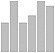vertical bar chart
BarChart[{7, 3, 8, 5, 5},
ChartLegends->
{"a","b","c","d","e"}]
x: [7, 3, 8, 5, 5];
labs: [a, b, c, d, e];
data: makelist(makelist(labs[i], j, x[i]), i, 5);
wxbarsplot(flatten(data));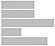horizontal bar chart
BarChart[{7, 3, 8, 5, 5}, BarOrigin -> Left] none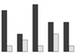grouped bar chart
data = {{7, 1}, {3, 2}, {8, 1}, {5, 3}, {5, 1}}
BarChart[data]
x: [7, 3, 8, 5, 5];
y: [1,2,1,3,1];
labs: [a, b, c, d, e];
d1: makelist(makelist(labs[i], j, x[i]), i, 5);
d2: makelist(makelist(labs[i], j, y[i]), i, 5);
wxbarsplot(flatten(d1), flatten(d2));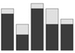stacked bar chart
data = {{7, 1}, {3, 2}, {8, 1}, {5, 3}, {5, 1}}
BarChart[data, ChartLayout -> "Stacked"]
x: [7, 3, 8, 5, 5];
y: [1,2,1,3,1];
labs: [a, b, c, d, e];
d1: makelist(makelist(labs[i], j, x[i]), i, 5);
d2: makelist(makelist(labs[i], j, y[i]), i, 5);
wxbarsplot(flatten(d1), flatten(d2),
grouping=stacked);pie chart
PieChart[{7, 3, 8, 5, 5}] x: [7, 3, 8, 5, 5];
labs: [a, b, c, d, e];
data: makelist(makelist(labs[i], j, x[i]), i, 5);
wxpiechart(flatten(data));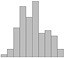histogram
X = NormalDistribution[0, 1]
(* 2nd arg is approx number of bins: *)
Histogram[RandomReal[X, 100], 10]

data: makelist(random_normal(0, 1), i, 1, 100);
wxhistogram(data);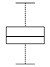box plot
X = NormalDistribution[0, 1]
n100 = RandomVariate[X, 100]
BoxWhiskerChart[n100]

Y = ExponentialDistribution
e100 = RandomVariate[Y, 100]
u100 = RandomReal[1, 100]
data = {n100, e100, u100}
BoxWhiskerChart[data]

data: makelist(random_normal(0, 1), i, 1, 100);
wxboxplot(data);
scatter plots
mathematica sympy sage maxima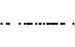strip chart
X = NormalDistribution[0, 1]
data = {RandomReal[X], 0} & /@ Range[1, 50]
ListPlot[data]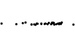strip chart with jitter
X = NormalDistribution[0, 1]
Y = UniformDistribution[{-0.05, 0.05}]
data = {RandomReal[X], RandomReal[Y]} & /@
Range[1, 50]
ListPlot[data,
PlotRange -> {Automatic, {-1, 1}}]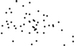scatter plot
X = NormalDistribution[0, 1]
rand = Function[RandomReal[X]]
data = {rand[], rand[]} & /@ Range[1, 50]
ListPlot[data]

x: makelist(random_normal(0, 1), i, 1, 50);
y: makelist(random_normal(0, 1), i, 1, 50);
wxplot2d([discrete, x, y], [style, points]);X = NormalDistribution[0, 1]
rand = Function[RandomReal[X]]
data1 = {rand[], rand[]} & /@ Range[1, 50]
data2 = {rand[]+1, rand[]+1} & /@ Range[1, 50]
Show[ListPlot[data1],
ListPlot[data2, PlotStyle -> Red]]

x1: makelist(random_normal(0, 1), i, 1, 50);
y1: makelist(random_normal(0, 1), i, 1, 50);
x2: makelist(random_normal(0, 1), i, 1, 50) + 1;
y2: makelist(random_normal(0, 1), i, 1, 50); + 1;
wxplot2d([[discrete, x1, y1],
[discrete, x2, y2]],
[style, points], [color, black, red]);
point types ListPlot[data, PlotMarkers -> {"*"}]

(* shows standard sequence of point types: *)
GraphicsPlotMarkers[]

(* The elements of the PlotMarkers array can be strings, symbols, expressions, or images. *)
wxplot2d([discrete, x, y],
[style, points],
[point_type, asterisk]);

/* possible point_type values:

asterisk
box
bullet
circle
diamond
plus
square
times
triangle

The bullet and box are filled versions of circle and square.
*/
point size X = NormalDistribution[0, 1]
rand = Function[RandomReal[X]]
data = {rand[], rand[]} & /@ Range[1, 50]
(* point size is fraction of plot width: *)
ListPlot[data, PlotStyle -> {PointSize[0.03]}]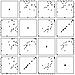scatter plot matrix
Needs["StatisticalPlots"]

X = NormalDistribution[0, 1]
x = RandomReal[X, 50]
y = RandomReal[X, 50]
z = x + 3 * y
w = y + RandomReal[X, 50]
PairwiseScatterPlot[Transpose[{x, y, z, w}]]

x: makelist(random_normal(0, 1), i, 1, 50);
y: makelist(random_normal(0, 1), i, 1, 50);
z: x + 3 * y;
w: y + makelist(random_normal(0, 1), i, 1, 50);
wxscatterplot(transpose(matrix(x, y, z, w)));3d scatter plot
X = NormalDistribution[0, 1]
data = RandomReal[X, {50, 3}]
ListPointPlot3D[data]
none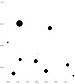bubble chart
X = NormalDistribution[0, 1]
data = RandomReal[X, {50, 3}]
BubbleChart[data]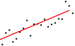linear regression line
data = Table[{i, 2 * i + RandomReal[{-5, 5}]},
{i, 0, 20}]
model = LinearModelFit[data, x, x]
Show[ListPlot[data],
Plot[model["BestFit"],
{x, 0, 20}]]

X: makelist(i, i, 50);
Y: makelist(X[i] + random_normal(0, 1), i, 50);
M: transpose(matrix(X, Y));
fit: lsquares_estimates(M, [x, y], y = A*x + B,
[A, B]);
A: second(fit), numer;
B: second(fit), numer;
Xhat: makelist(A*X[i] + B, i, 50);
wxplot2d([[discrete, X, Y], [discrete, X, Xhat]],
[style, points, lines], [color, black, red]);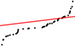quantile-quantile plot
X = NormalDistribution[0, 1]
data1 = RandomReal[1, 50]
data2 = RandomReal[X, 50]
QuantilePlot[data1, data2]

x: makelist(random_continuous_uniform(0, 1),
i, 200);
y: makelist(random_normal(0, 1), i, 200);
wxplot2d([discrete, sort(x), sort(y)],
[style, points]);
line charts
mathematica sympy sage maxima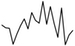polygonal line plot
X = NormalDistribution[0, 1]
rand = Function[RandomReal[X]]
f = Function[i, {i, rand[]}]
data = f /@ Range[1, 20]
ListLinePlot[data]

x: makelist(random_normal(0, 1), i, 1, 20);
wxplot2d([discrete, makelist(i, i, 20), x]);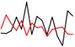X = NormalDistribution[0, 1]
data1 = RandomReal[X, 20]
data2 = RandomReal[X, 20]
ListLinePlot[{data1, data2}
PlotStyle->{Black, Red}]

x: makelist(random_normal(0, 1), i, 1, 20);
y: makelist(random_normal(0, 1), i, 1, 20);
wxplot2d([[discrete, makelist(i, i, 20), x],
[discrete, makelist(i, i, 20), y]],
[color, black, red]);
line types ListLinePlot[data, PlotStyle -> Dashed]

(* PlotStyle values:

Dashed
DotDashed
Dotted
*)
none
line thickness X = NormalDistribution[0, 1]
data1 = RandomReal[X, 20]
data2 = RandomReal[X, 20]
(* thickness is fraction of plot width: *)
ListLinePlot[{data1, data2},
PlotStyle -> {Thickness[0.01], Thickness[0.02]}]function plot
Plot[Sin[x], {x, -4, 4}] wxplot2d(sin(x), [x, -4, 4]);parametric plot
ParametricPlot[{Sin[u], Sin[2 * u]},
{u, 0, 2 * Pi}]
wxplot2d([parametric, sin(t), sin(2*t),
[t, 0, 2*%pi]]);implicit plot
ContourPlot[x^2 + y^2 == 1, {x, -1, 1},
{y, -1, 1}]

wximplicit_plot(x^2 + y^2 = 1, [x, -1, 1],
[y, -1, 1]);polar plot
PolarPlot[Sin[3 * t], {t, 0, Pi}] f(x) := sin(3 * x);
wxplot2d([parametric, cos(t)*f(t), sin(t)*f(t),
[t, 0, %pi]]);cubic spline
X = NormalDistribution[0, 1]
data = Table[{i, RandomReal[X]},
{i, 0, 20}]
f = Interpolation[data,
InterpolationOrder -> 3]
Show[ListPlot[data],
Plot[f[x], {x, 0, 20}]]

data: makelist([i, random_normal(0, 1)], i, 20);
cspline(data);
f(x):=''%;
wxdraw2d(explicit(f(x),x,0,20));area chart
data = {{7, 1, 3, 2, 8}, {1, 5, 3, 5, 1}}
stacked = {data[], data[] + data[]}
ListLinePlot[stacked, Filling ->
{1 -> {Axis, LightBlue},
2 -> {{1}, LightRed}}]
surface charts
mathematica sympy sage maximacontour plot
(* of function: *)
ContourPlot[x * (y - 1), {x, 0, 10},
{y, 0, 10}]

(* of data: *)
X = NormalDistribution[0, 1]
rand = Function[RandomReal[X]]
data = Table[x * (y - 1) + 5 * rand[],
{x, 0, 10}, {y, 0, 10}]
ListContourPlot[data]
wxcontour_plot(x * (y-1), [x, 0, 10],
[y, 0, 10]);heat map
(* of function: *)
DensityPlot[Sin[x] * Sin[y],
{x, -4, 4},
{y, -4, 4}]

(* of data: *)
X = NormalDistribution[0, 1]
rand = Function[RandomReal[X]]
data = Table[x * y + 10 * rand[],
{x, 1, 10},
{y, 1, 10}]
ListDensityPlot[data]
wxplot3d (sin(x) * sin(y), [x,-4,4], [y,-4,4],
[elevation, 0], [azimuth, 0],
[grid, 100, 100], [mesh_lines_color, false]);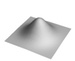Plot3D[Exp[-(x^2 + y^2)], {x, -2, 2},
{y, -2, 2}, MeshStyle -> None]
light source lot3D[Exp[-(x^2 + y^2)],
{x, -2, 2}, {y, -2, 2},
MeshStyle -> None,
Lighting -> {{"Point", White, {5, -5, 5}}}]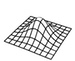mesh surface plot
Plot3D[Exp[-(x^2 + y^2)], {x, -2, 2},
{y, -2, 2}, Lighting -> {White},
PlotStyle -> White]
wxplot3d(exp(-(x^2 + y^2)),
[x, -2, 2], [y, -2, 2],
[palette, false], [color, black]);
view point (* (x, y, z) coordinates;
(0, 0, 3) is from above: *)

Plot3D[Exp[-(x^2 + y^2)],
{x, -2, 2}, {y, -2, 2},
MeshStyle -> None,
ViewPoint -> {0, 0, 3}]vector field plot
StreamPlot[{x^2 + y, 1 + x - y^2}, {x, -4, 4}, {y, -4, 4}] plotdf([x^2 + y, 1 + x - y^2], [x, -4, 4],
[y, -4, 4]);
chart options
mathematica sympy sage maxima
chart title (* title on top by default *)
Plot[Sin[x], {x, -4, 4},
PlotLabel -> "title example"]
wxplot2d(sin(x), [x, -4, 4],
[title, "title example"]);

data: 1, 1, 2, 2, 3, 3, 3, 3, 4];
wxboxplot(data, title="title example");

/* title on top by default */
axis label data = Table[{i, i^2}, {i, 1, 20}]
ListLinePlot[data, AxesLabel -> {x, x^2}]
x: makelist(i, i, 20);
y: makelist(i^2, i, 20);
wxplot2d([discrete, x, y],
[xlabel, "x"], [ylabel, "x^2"]);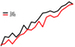legend
X = NormalDistribution[0, 1]
data1 = RandomReal[X, 20]
data2 = RandomReal[X, 20]
ListLinePlot[{data1, data2},
PlotLegends -> {"first", "second"}]
/* wxplot2d includes a legend by default.

Provide [legend, false] as argument to suppress it. */
data label data = {{313, 3.7}, {62, .094}, {138, 6.6},
{113, 0.76}, {126, 0.15}}

(* The {0, -1} argument of Text[] centers the label above the data point. *)
Show[ListPlot[data,
AxesLabel -> {"pop", "area"}],
Graphics[Text["USA", data[], {0, -1}]],
Graphics[Text["UK", data[], {0, -1}]],
Graphics[Text["Russia", data[], {0, -1}]],
Graphics[Text["Mexico", data[], {0, -1}]],
Graphics[Text["Japan", data[], {0, -1}]]]
named colors White Gray Black Transparent

Blue Brown Cyan Green Magenta Orange Pink Purple Red Yellow

LightBlue LightBrown LightCyan LightGray LightGreen LightMagenta LightOrange LightPink LightPurple LightRed LightYellow
white gray black

gray0 gray10 gray100 gray20 gray30 gray40 gray50 gray60 gray70 gray80 gray90 gray100 grey grey0 grey10 grey20 grey30 grey40 grey50 grey60 grey70 grey80 grey90 grey100

aquamarine beige blue brown coral cyan forest_green gold goldenrod green khaki magenta medium_blue midnight_blue navy orange orange_red pink plum purple red royalblue salmon sea_green skyblue spring_green turquoise violet yellow

dark_blue dark_cyan dark_goldenrod dark_gray dark_green dark_grey dark_khaki dark_magenta dark_orange dark_pink dark_red dark_salmon dark_turquoise dark_violet dark_yellow

light_blue light_coral light_cyan light_goldenrod light_gray light_green light_grey light_magenta light_pink light_red light_salmon light_turquoise light_yellow
rgb color RGBColor[1, 0, 0]
(* with opacity: *)
RGBColor[1, 0, 0, 0.5]
[color, "#FF0000"]
background color Plot[Sin[x], {x, 0, 2 Pi},
Background -> Black,
PlotStyle -> White,
AxesStyle -> White,
TicksStyle -> White,
GridLines -> Automatic,
GridLinesStyle -> White]
axis limits Plot[x^2, {x, 0, 20},
PlotRange -> {{0, 20}, {-200, 500}}]
logarithmic y-axis LogPlot[{x^2, x^3, x^4, x^5},
{x, 0, 20}]
x: makelist(i, i, 20);
wxplot2d([
[discrete, x, makelist(i^2, i, 20)],
[discrete, x, makelist(i^3, i, 20)]],
[logy, true]);
aspect ratio (* aspect ratio is height divided by width: *)
Plot[Sin[x], {x, 0, 2 Pi}, AspectRatio -> 0.25]

(* In the notebook, dragging the corner of an image increases or decreases the size, but aspect ratio is preserved. *)
wxplot2d(sin(x), [x, -4, 4],
[yx_ratio, 0.25]);

/* Image size can’t be changed in notebook. */
ticks Plot[Sin[x], {x, 0, 2 Pi}, Ticks -> None]

Plot[Sin[x], {x, 0, 2 Pi},
Ticks -> {{0, Pi, 2*Pi}, {-1, 0, 1}}]
wxplot2d(sin(x), [x, -4, 4],
[xtics, -4, 2, 4],
[ytics, -1, 0.5, 1]);
grid lines Plot[Sin[x], {x, 0, 2 Pi},
GridLines -> Automatic]

Plot[Sin[x], {x, 0, 2 Pi},
GridLines -> {{0, 1, 2, 3, 4, 5, 6},
{-1, -0.5, 0, 0.5, 1}}]grid of subplots
GraphicsGrid[Table[Table[
Histogram[RandomReal[X, 100], 10],
{i, 1, 2}],
{j, 1, 2}]]

x: makelist(makelist(random_normal(0, 1), i, 50),
j, 4);
p: makelist(histogram_description(x[i]), i, 4);
wxdraw(gr2d(p), gr2d(p), gr2d(p),
gr2d(p), columns=2);
save plot as png Export["hist.png",
Histogram[RandomReal[X, 100], 10]]
After creating a plot, run gnuplot on the .gnuplot file generated in the home directory:

gnuplot maxout.gnuplot ____________________________________________________ ____________________________________________________ ____________________________________________________ ____________________________________________________ # Symbolic Expressions In many programming languages, attempting to evaluate an expression with an undefined variable results in an error. Some languages assign a default value to variables so that such expressions can be evaluated. In a CAS, undefined variables are treated as unknowns; expressions which contains them are symbolic expressions. When evaluating them, if the unknowns cannot be eliminated, the expression cannot be reduced to a numeric value. The expression then evaluates to a possibly simplified or normalized version of itself. Symbolic expressions are first class values; they can be stored in variables or passed to functions. An application of symbolic expressions is a function which solves a system of equations. Without symbolic expressions, it would be awkward for the caller to specify the equations to be solved. ## literal How to create a symbolic expression. In most CAS systems, any expression an undefined variables is automatically a a symbolic expression. sympy: In SymPy, unknowns must be declared. This is a consequence of SymPy being implemented as a library in a language which throws exceptions when undefined variables are encountered. ## prevent simplification ## variable update Do symbolic expressions "see" changes to the unknown variables they contain. ## substitute ## piecewise-defined expression ## simplify ## assumption ## assumption predicates ## list assumptions ## remove assumption # Calculus ## limit ## limit at infinity ## one-sided limit ## derivative ## derivative of a function ## constants ## higher order derivative ## mixed partial derivative ## div, grad, and curl ## antiderivative ## definite integral ## improper integral ## double integral ## find poles ## residue ## sum ## series sum ## series expansion of function ## omitted order term ## product # Equations and Unknowns ## solve equation ## solve equations ## differential equation ## differential equation with boundary condition ## differential equations ## recurrence equation # Optimization An optimization problem consists of a real-valued function called the objective function. The objective function takes one or more input variables. In the case of a maximization problem, the goal is to find the value for the input variables where the objective function achieves its maximum value. Similarly for a minimization function one looks for the values for which the objective function achieves its minimum value. ## minimize How to solve a minimization problem in one variable. ## maximize How to solve a maximization problem. We can use a function which solves minimization problems to solve maximization problems by negating the objective function. The downside is we might forget the minimum value returned is the negation of the maximum value we seek. ## objective with unknown parameter How to solve an optimization when the objective function contains unknown parameters. ## unbounded behavior What happens when attempting to solve an unbounded optimization problem. ## multiple variables How to solve an optimization problem with more than one input variable. ## constraints How to solve an optimization with constraints on the input variable. The constrains are represented by inequalities. ## infeasible behavior What happens when attempting to solve an optimization problem when the solution set for the constraints is empty. ## integer variables How to solve an optimization problem when the input variables are constrained to linear values. # Vectors ## vector literal The notation for a vector literal. ## constant vector How to create a vector with components all the same. ## vector coordinate How to get one of the coordinates of a vector. ## vector dimension How to get the number of coordinates of a vector. ## element-wise arithmetic operators How to perform an element-wise arithmetic operation on vectors. ## vector length mismatch What happens when an element-wise arithmetic operation is performed on vectors of different dimension. ## scalar multiplication How to multiply a scalar with a vector. ## dot product How to compute the dot product of two vectors. ## cross product How to compute the cross product of two three-dimensional vectors. ## norms How to compute the norm of a vector. # Matrices ## literal or constructor Literal syntax or constructor for creating a matrix. mathematica: Matrices are represented as lists of lists. No error is generated if one of the rows contains too many or two few elements. The MatrixQ predicate can be used to test whether a list of lists is matrix: i.e. all of the sublists contain numbers and are of the same length. Matrices are displayed by Mathematica using list notation. To see a matrix as it would be displayed in mathematical notation, use the MatrixForm function. ## construct from sequence ## constant matrices ## diagonal matrices ## matrix by formula ## dimensions How to get the number of rows and columns of a matrix. ## element lookup How to access an element of a matrix. The anguages described here follow the mathematical convention of putting the row index before the column index. ## extract row How to access a row. ## extract column How to access a column. ## extract submatrix How to access a submatrix. ## scalar multiplication How to multiply a matrix by a scalar. ## element-wise operators Operators which act on two identically sized matrices element by element. Note that element-wise multiplication of two matrices is used less frequently in mathematics than matrix multiplication. ## product How to multiply matrices. Matrix multiplication in non-commutative and only requires that the number of columns of the matrix on the left match the number of rows of the matrix. Element-wise multiplication, by contrast, is commutative and requires that the dimensions of the two matrices be equal. ## power How to compute the power of a square matrix. For non-negative integers, the power of a matrix is defined recursively with A0 = I and An = An-1 ⋅ A. If the matrix is invertible, the power is defined for negative integers by An = (A-1)-n. ## exponential (1) \begin{align} \exp(A) = \sum_{i=0}^\infty A^i \end{align} ## log ## kronecker product The Kronecker product is a non-commutative operation defined on any two matrices. If A is m x n and B is p x q, then the Kronecker product is a matrix with dimensions mp x nq. ## norms How to compute the 1-norm, the 2-norm, the infinity norm, and the frobenius norm. ## transpose ## conjugate transpose ## inverse ## row echelon form ## pseudoinverse ## determinant The determinant of a square matrix is equal to the product of the eigenvalues of a matrix. It is zero if and only if the matrix is nonsingular. The determinant can be computed by calculating the matrix cofactors along a row or column, multiplying each by the row or column entry, and then summing. This technique, called Laplace's formula, requires n! multiplications, where n is number of rows in matrix, and thus is impractical for large matrices. ## trace The trace of a matrix is the sum of the diagonal entries. It is equal to the sum of the eigenvalues of the matrix. ## characteristic polynomial The charateristic polynomial of a matrix A can be defined by: (2) \begin{align} p(t) = \mathrm{det}(t ⋅ I - A) \end{align} The eigenvalues of A are the roots of p(t). ## minimal polynomial ## rank ## nullspace basis ## eigenvalues ## eigenvectors ## LU decomposition A factorization into a lower triangular matrix L, an upper triangular matrix U, and a permutation matrix P. Typically the identity is PA = LU. An LU factorization of a square matrix always exists. It can be found using a modified variant of Gaussian elimination. For an n × n matrix it requires about n3 scalar multiplications. LU factorization is an efficient way (1) to solve a system of equations, (2) to find the inverse of a matrix, and (3) to compute the determinant of a matrix. To use PA = LU to solve Ax = b, first solve for y in Ly = Pb using forward substitution, then solve for x in Ux = y using backward substitution. To use PA = LU to find the inverse B of A, first solve for Y in LU = P using forward substitution, then solve for UB = Y using backward substitution. The determinant of A can be computed from the diagonal entries of L and U. To get the sign correctly, one must count the number of row exchanges S in the permutation matrix P: (3) \begin{align} (-1)^S \prod_{i=1}^n l_{ii} \prod_{i=1}^n u_{ii} \end{align} ## QR decomposition A factorization of a square matrix into an orthogonal matrix Q and an upper triangular matrix R. The QR factorization is unique when the original matrix A is invertible. The Gram-Schmidt process can be used to compute a QR factorization, though it is not the most numerically stable method. If a1, …, an are the column vectors of the original matrix A, then the Gram-Schmidt process yields the column vectors e1, …, en of the orthogonal matrix Q. The QR algorithm uses QR factorizations to iteratively find eigenvalues. For each iteration we perform a QR factorization on Ak: (4) \begin{equation} Q_k R_k = A_k \end{equation} Then we multiply Q_k and R_k in reverse to get Ak+1: (5) \begin{equation} A_{k+1} = R_k Q_k \end{equation} Usually the sequence of Ak will converge to a triangular matrix with the eigenvalues on the diagonal. The limit matrix is similar to the original matrix because (6) \begin{equation} A_{k+1} = R_k Q_k = R_k A_k R_k^{-1} \end{equation} and hence has the same eigenvalues. ## spectral decomposition The spectral decomposition of a square matrix A is a factorization P ⋅D ⋅ P-1 where P is invertible and D is diagonal. The spectral decomposition is also called the eigendecomposition. The values on the diagonal of D are eigenvalues of the matrix A and the rows of P are eigenvectors. If a spectral decomposition exists, the matrix A is said to be diagonalizable. If an invertible matrix P exists such that A = P ⋅ B ⋅ P-1, then A and B are said to be similar. According to the spectral theorem, a spectral decomposition exists when the matrix A is normal, which means it commutes with its conjugate transpose. If a matrix A is symmetric, then a spectral decomposition P ⋅ D ⋅ P-1 exists, and moreover P and D are real matrices. ## singular value decomposition A singluar value decomposition of a matrix A is a factorization into a diagonal matrix S and unitary matrices U and V such that A = U ⋅ S ⋅ V*. Unlike the spectral decomposition, an SVD always exists, even if A is not square. The values on the diagonal of S are called the singular values, and they are the eigenvalues of A ⋅ A*. ## jordan decomposition The Jordan decomposition of a square matrix A is a factorization A = P ⋅ J ⋅ P-1 where J is in Jordan canonical form. ## polar decomposition A factorization of a square matrix into a unitary matrix U and a positive definite Hermitian matrix P. All invertible matrices have a polar decomposition. A unitary matrix corresponds to a linear transformation representing a rotation, reflection, or a combination of the two. It is distance perserving, in that it maps vectors to vectors of the same length. A real valued unitary matrix is called an orthogonal matrix. # Combinatorics Enumerative combinatorics is the study of the size of finite sets. The sets are defined by some property, and we seek a formula for the size of the set so defined. For some simple examples, let A and B be disjoint sets of size n and m respectively. The size of the union A ∪ B is n + m and the size of the Cartesian product A × B is nm. The size of the power set of A is 2n. ## factorial The factorial function n! is the product of the first n positive integers 1 × 2 × … × n. It is also the number of permutations or bijective functions on a set of n elements. It is the number of orderings that can be given to n elements. See the section on permutations below for how to iterate through all n! permutations on a set of n elements. As the factorial function grows rapidly with n, it is useful to be aware of this approximation: (7) \begin{align} \ln n! \approx n \ln n - n + \frac{1}{2} \ln 2 \pi n \end{align} ## binomial coefficient A binomial coefficient can be computed using the factorial function: (8) \begin{align} {n \choose k} = \frac{n!}{(n-k)! k!} \end{align} The binomial coefficient appears in the binomial theorem: (9) \begin{align} (x+y)^n = \sum_{k=0}^{n} {n \choose k} x^k y^{n-k} \end{align} The binomial cofficient{ n \choose k }is the number of sets of size k which can be drawn from a set of size n without replacement. ## multinomial coefficient The multinomial coefficient generalizes the binomial cofficient: (10) \begin{align} {n \choose k_1, \ldots, k_m} = \frac{n!}{(k_1! \cdots k_m!} \end{align} It appears in the multinomial theorem: (11) \begin{align} (x_1 + \cdots + x_m)^n = \sum_{k_1 + \cdots + k_m = n} {n \choose k_1, \ldots, k_m} \prod_{t=1}^m x_t^{k_t} \end{align} The multinomial cofficient{ n \choose k_1,ldots,k_m }is the number of ways to partition a set of n elements into subsets of size k1, …, km where the ki sum to n. ## rising and falling factorial ## subfactorial A derangement is a permutation on a set of n elements where every element moves to a new location. The number of derangements is thus less than the number of permutations, n!, and the function for the number of derangements is called the subfactorial function. Using a exclamation point as a prefix to denote the subfactorial, the following equations hold: (12) \begin{align} !n = n \cdot [!(n-1)] + (-1)^n \end{align} (13) \begin{align} !n = n! \sum_{i=0}^n \frac{(-1)^i}{i!} \end{align} (14) \begin{align} lim_{n \rightarrow \infty} \frac{!n}{n!} = \frac{1}{e} \end{align} ## integer partitions The number of multisets of positive integers which sum to a integer. There are 5 integer partitions of 4:  4 3 + 1 2 + 2 2 + 1 + 1 1 + 1 + 1 + 1  ## compositions The number of sequences of positive integers which sum to an integer. There are 8 compositions of 4:  4 3 + 1 1 + 3 2 + 2 2 + 1 + 1 1 + 2 + 1 1 + 1 + 2 1 + 1 + 1 + 1 ` mathematica: The NumberOfCompositions and Compositions functions use weak compositions, which include zero as a possible summation. The number of weak compositions of an integer is infinite, since there is no limit on the number of times zero can appear as a summand. The number of weak compositions of a fixed size is finite, however. ## set partitions ## bell number ## permutations with k disjoint cycles ## fibonacci number ## bernoulli number ## harmonic number ## catalan number # Number Theory ## divisible test A test whether an integer a is divisible by another integer b. Equivalently, does there exists a third integer m such that a = mb. ## divisors The list of divisors for an integer. ## pseudoprime test A fast primality test. An integer p is prime if for any factorization p = ab, where a and b are integers, either a or b are in the set {-1, 1}. A number of primality tests exists which give occasional false positives. The simplest of these use Fermat's Little Theorem, in which for prime p and a in\{1, ..., p - 1\}: (15) \begin{align} a^{p-1} \equiv 1 \;(\text{mod}\; p) \end{align} The test for a candidate prime p is to randomly choose several values for a in\{1, ..., p - 1\}and evaluate (16) \begin{align} a^{p-1} \;(\text{mod}\; p) \end{align} If any of them are not equivalent to 1, then the test shows that p is not prime. Unfortunately, there are composite numbers n, the Carmichael numbers, for which (17) \begin{align} a^{n-1} \equiv 1 \;(\text{mod}\; n) \end{align} holds for all a in\{1, ..., n - 1\}. A stronger test is the Miller-Rabin primality test. Given a candidate prime n, we factor n - 1 as 2rd where d is odd. If n is prime, then one of the following must be true: (18) \begin{align} a^d \equiv 1 \;(\text{mod}\;n) \end{align} (19) \begin{align} a^{2^r \cdot d} \equiv -1 \;(\text{mod}\;n) \end{align} Thus, one checks the above two equations for a small number of primes. If we use all primes p ≤ 41, then it is known that there are no false positives for n ≤ 3 × 1024. Since pseuodoprime tests are known which are correct for all integers up to a very large size, and since conclusively showing that a number is prime is a slow operation for larger integers, a true prime test is often not practical. ## prime factors The list of prime factors for an integer, with their multiplicities. ## next prime The smallest prime number greater than an integer. Also the greatest prime number smaller than an integer. ## nth prime The n-th prime number. ## prime counting function The number of primes less than or equal to a value. According to the prime number theorem: (20) \begin{align} \lim_{n \rightarrow \infty} \frac{\pi(n)}{n/\log n} = 1 \end{align} ## divmod The quotient and remainder. If the divisor is positive, then the remainder is non-negative. ## greatest common divisor The greatest common divisor of a pair of integers. The divisor is always positive. Two integers are relatively prime if their greatest common divisor is one. ## extended euclidean algorithm How to express a greatest common divisor as a linear combination of the integers it is a GCD of. The functions described return the GCD in addition to the coefficients. ## least common multiple The least common multiple of a pair of integers. The LCM can be calculated from the GCD using this formula: (21) \begin{align} \text{lcm}(m, n) = \frac{|m\cdot n|}{\text{gcd}(m, n)} \end{align} ## power modulus Raise an integer to a integer power, modulo a third integer. Euler's theorem can often be used to reduce the size of the exponent. ## integer residues The integer residues or integers modulo n are the equivalence classes formed by the relation (22) \begin{align} a\;(\text{mod}\;n) = b\; (\text{mod}\;n) \end{align} An element in of these equivalence classes is called a representative. We can extend addition and multiplication to the residues by performing integer addition or multiplication on representatives. This is well-defined in the sense that it does not depend on the representatives chosen. Addition and multiplication defined this way turn the integer residues into commutative rings with identity. ## multiplicative inverse How to get the multiplicative inverse for a residue. If the representative for a residue is relatively prime to the modulus, then the residue has a multiplicative inverse. For that matter, if the modulus n is a prime, then the ring of residues is a field. Note that we cannot in general find the inverse using a representative, since the only units in the integers are -1 and 1. By Euler's theorem, we can find a multiplicative inverse by raising it to the power\phi(n) - 1: (23) \begin{align} a^{\phi(n) - 1} \cdot a = a^{\phi(n)} \equiv 1 \;(\text{mod}\;n) \end{align} When a doesn't have a multiplicative inverse, then we cannot cancel it from both sides of a congruence. The following is true, however: (24) \begin{align} az \equiv az' \;(\text{mod}\; n) \iff z \equiv z' \;\left(\text{mod}\; \frac{n}{\text{gcd}(a, n)}\right) \end{align} ## chinese remainder theorem A function which finds a solution to a system of congruences. The Chinese remainder theorem asserts that there is a solution x to the system of k congruences (25) \begin{align} x \equiv a_i \;(\text{mod}\;n_i) \end{align} provided that the ni are pairwise relatively prime. In this case there are an infinite number of solutions, all which are equal moduloN = n_1 \cdots n_k$. For this reason the solution is returned as a residue modulo N. ## lift integer residue How to get a representative from the equivalence class of integers modulo n. Typically an integer in$\{0, ..., n - 1\}$is chosen. A centered lift chooses a representative x such that$-n/2 < x \leq n/2. ## euler totient The Euler totient function is defined for any positive integer n as: (26) \begin{align} \phi(n) = n \prod_{p | n} \frac{p - 1}{p} \end{align} Note that the product is over the primes that divide n. The Euler totient is the number of integers in\{1, ..., n - 1\}\$ which are relatively prime to n. It is thus the size of the multiplicative group of integers modulo n.

The Euler totient appears in Euler's theorem:

(27)
\begin{align} a^{\phi(n)} \equiv 1 \;(\text{mod}\;n) \end{align}

## carmichael function

The smallest number k such that ak ≡ 1 (mod n) for all residues a.

By Euler's theorem, the Carmichael function λ(n) is less that or equal to the Euler totient function φ(n). The functions are equal when there are primitive roots modulo n.

## multiplicative order

The multiplicative order of a residue a is the smallest exponent k such that

(28)
\begin{align} a^k \equiv 1\;(\text{mod}\;n) \end{align}

In older literature, it is sometimes said that a belongs to the exponent k modulo n.

## primitive roots

A primitive root is a residue module n with multiplicative order φ(n).

The multiplicative group is not necessarily cyclic, though it is when n is prime. If it is not cyclic, then there are no primitive roots.

Any primitive root is a generator for the multiplicative group, so it can be used to find the other primitive roots.

## discrete logarithm

For a residue x and a base residue b, find a positive integer such that:

(29)
\begin{align} b^k \equiv x\;(\text{mod}\; n) \end{align}

A quadratic residue is a non-zero residue a which has a square root modulo p. That is, there is x such that

(30)
\begin{align} x^2 \equiv a \;(\text{mod}\;p) \end{align}

If a is non-zero and doesn't have a square root, then it is a quadratic non-residue.

## discrete square root

How to find the square root of a quadratic residue.

## kronecker symbol

The Legendre symbol is used to indicate whether a number is a quadratic residue and is defined as follows:

(31)
\begin{align} \left( \frac{a}{p} \right) = \begin{cases} \;\; 1 \;\;\; a \; \text{is a quadratic residue} \\ \;\; 0 \;\;\; p \mid a \\ -1 \;\;\; a \; \text{is a quadratic nonresidue} \end{cases} \end{align}

The Legendre symbol is only defined when p is an odd prime, but if n is an odd positive integer with prime factorization

(32)
\begin{align} p_1^{\alpha_1} \ldots p_n^{\alpha_n} \end{align}

then the Jacobi symbol is defined as

(33)
\begin{align} \left( \frac{a}{n} \right) = \left( \frac{a}{p_1} \right)^{\alpha_1} \ldots \left( \frac{a}{p_n} \right)^{\alpha_n} \end{align}

The Kronecker symbol is a generalization of the Jacobi symbol to all integers, but we omit the details.

## moebius function

The Möbius function μ(n) is 1, -1, or 0 depending upon when n is a square-free integer with an even number of prime factors, a square-free integer with an odd number of prime factors, or an integer which is divisible by p2 for some prime p.

The Möbius function is multiplicative: when a and b are relatively prime, μ(a)μ(b) = μ(ab).

The Möbius function appears in the Möbius inversion formula. If g and f are possibly complex-valued functions defined on the natural numbers such that for all integers n ≥ 1:

(34)
\begin{align} g(n) = \sum_{d | n} f(d) \end{align}

then for all integers n ≥ 1:

(35)
\begin{align} f(n) = \sum_{d | n} \mu(d) g(n | d) \end{align}

## riemann zeta function

The Riemann zeta function is a complex-valued function defined as the analytic continuation of this series:

(36)
\begin{align} \zeta(s) = \sum_{n=1}^\infty \frac{1}{n^s} \end{align}

The function has zeros (called the trivial zeros) at -2, -4, …. All other zeros must lie in the strip 0 ≤ ℜ(z) ≤ 1. In 1859 Riemann conjectured that all non-trivial zeros are on the line ℜ(z) = 1/2.

## continued fraction

Convert a real number to a continued fraction.

A continued fraction is a sequence of integers a0, a1, …, an representing the fraction:

(37)
\begin{align} a_0 + \frac{1}{a_1 + \frac{1}{a_2 + \frac{1}{\ddots + {\frac{1}{a_n}}}}} \end{align}

The sequence can even be infinite, in which case the fraction is the limit of the rational numbers defined by taking the first n digits in the sequence.

A continued fraction for a real number can be computed using the Euclidean algorithm. In the case of a rational number, one starts with the numerator and the denominator. In the case of a rational number, one can start with the number itself and 1.

A continued fraction is finite if and only if the number is a rational.

A continued fraction repeats if and only if it is a quadratic irrational.

## convergents

The first n digits of a continued fraction define a sequence of rational numbers called the convergents. The rational numbers converge to the number defined by the continued fraction.

Each convergent r/q is the closest rational number to the continued fraction with denominator of size q or smaller.

# Polynomials

## factor

[[ #collect-terms-note]]

# Special Functions

## gamma function

The gamma function is defined for all complex numbers except the non-positive integers.

For positive integers, the following equation holds:

(38)
\begin{align} \Gamma(n) = (n-1)! \end{align}

If the real part of t is positive, then

(39)
\begin{align} \Gamma(t) = \int_0^\infty x^{t-1} e^{-x} dx \end{align}

## error function

The error function is function from ℝ to [-1, 1] defined by:

(40)
\begin{align} \mathrm{erf}(x) = \frac{2}{\sqrt(\pi)} \int_0^x e^{-t^2} dt \end{align}

The complementary error function is

(41)
\begin{align} \mathrm{erfc}(x) = 1 - erf(x) \end{align}

The cumulative distribution of the standard normal distribution is related to the error function by scaling:

(42)
\begin{align} \Phi(x) = \frac{1}{2} + \frac{1}{2} \mathrm{erf}(\frac{x}{\sqrt(2)}) = \frac{1}{2} \mathrm{erfc}(\frac{-x}{\sqrt(2)}) \end{align}

## hyperbolic functions

Definitions of the hyperbolic functions:

(43)
\begin{align} \mathrm{sinh}\;x = \frac{e^x - e^{-x}}{2} \end{align}
(44)
\begin{align} \mathrm{cosh}\;x = \frac{e^x + e^{-x}}{2} \end{align}
(45)
\begin{align} \mathrm{tanh}\;x = \frac{\mathrm{sinh}\;x}{\mathrm{cosh}\;x} \end{align}

sinh and cosh are odd and even functions, respectively. Like ex and e-x, sinh and cosh span the linear space of solutions to y''(x) = y(x).

# Permutations

A permutation is a bijection on a set of n elements.

The notation that Mathematica uses assumes the set the permutation operates on is indexed by {1, .., n}. The notation that SymPy uses assumes the set is indexed by {0, …, n - 1}.

Cayley two line notation

one line notation

cycle notation

inversions

## parity

Permutations are classified as even or odd based on the number of inversions.

The composition of two even permutations is even.

# Statistical Tests

A selection of statistical tests. For each test the null hypothesis of the test is stated in the left column.

In a null hypothesis test one considers the p-value, which is the chance of getting data which is as or more extreme than the observed data if the null hypothesis is true. The null hypothesis is usually a supposition that the data is drawn from a distribution with certain parameters.

The extremeness of the data is determined by comparing the expected value of a parameter according to the null hypothesis to the estimated value from the data. Usually the parameter is a mean or variance. In a one-tailed test the p-value is the chance the difference is greater than the observed amount; in a two-tailed test the p-value is the chance the absolute value of the difference is greater than the observed amount.

## wilcoxon signed-rank test

A non-parametric est whether a variable is drawn from a distribution that is symmetric about zero.

Often this test is used to test that the mean of the distribution is zero.

## kruskal-wallis rank sum test

A non-parametric test whether variables have the same mean.

For two variables, this test is the same as the Mann-Whitney test.

maxima:

The Maxima function only supports testing two variables.

## kolmogorov-smirnov test

Test whether two samples are drawn from the same distribution.

## one-sample t-test

Student's t-test determines whether a sample drawn from a normal distribution has mean zero.

The test can be used to test for a different mean value; just subtract the value from each value in the sample.

One may know in advance that the sample is drawn from a normal distribution. For example, if the values in the sample are each means of large samples, then the distribution is normal by the central limit theorem.

The Shapiro-Wilk test can be applied to determine if the values come from a normal distribution.

If the distribution is not known to be normal, the Wilcoxon signed-rank test can be used instead.

The Student's t-test used the sample to estimate the variance, and as a result the test statistic has a t-distribution.

By way of contrast, the z-test assumes that the variance is known in advance, and simply scales the data to get a z-score, which has standard normal distribution.

## independent two-sample t-test

Test whether two normal variables have same mean.

## paired sample t-test

A t-test used when the same individuals are measure twice.

## bartlett's test

A test whether variables are drawn from normal distributions with the same variance.

## levene's test

A test whether variables are drawn from distributions with the same variance.

# Bar Charts

## vertical bar chart

A chart in which the height of bars is used to represent a list of numbers.

maxima:

Maxima plots the frequency of the values, and not the values themselves. Non-positive values cannot be represented.

## horizontal bar chart

A bar chart in which zero is the y-axis and the bars extend to the right.

## pie chart

maxima:

Note that Maxima plots the frequency of the values, and not the values themselves.

## histogram

A histogram is a bar chart in which each bar represents the frequency of values in a data set within a range. The width of the bars can be used to indicate the ranges.

# Scatter Plots

## strip chart

A strip chart represents a list of values by points on a line. The values are converted to pairs by assigning the y-coordinate a constant value of zero. Pairs are then displayed with a scatter plot.

## strip chart with jitter

A strip chart in which in which a random variable with small range is used to fill the y-coordinate. Jitter makes it easier to see how many values are in dense regions.

## scatter plot

How to plot a list of pairs of numbers by representing the pairs as points in the (x, y) plane.

How to add a second list of pairs of numbers to a scatter plot. Color can be used to distinguish the two data sets.

## point types

How to select the symbols used to mark data points. Choice of symbols can be use to distinguish data sets.

## point size

How to change the size of the symbols used to mark points.

## scatter plot matrix

A scatter plot matrix is a way of displaying a multivariate data set by means of a grid of scatter plots. Off-diagonal plots are scatter plots of two of the variables. On-diagonal plots can be used to to display the name or a histogram of one of the variables.

## 3d scatter plot

How to represent a list of triples of numbers by points in (x, y, z) space.

## bubble chart

How to represent a list of triples of numbers by position in the (x, y) plane and size of the point marker.

It is probably better to associate the 3rd component of each triple with the area, and not the diameter of the point marker, but in general bubble charts suffer from ambiguity.

## linear regression line

How to add a linear regression line to a scatter plot.

# SymPy

Welcome to SymPy’s documentation!

# Sage

http://doc.sagemath.org/html/en/index.html

# Maxima

http://maxima.sourceforge.net/docs/manual/maxima.html